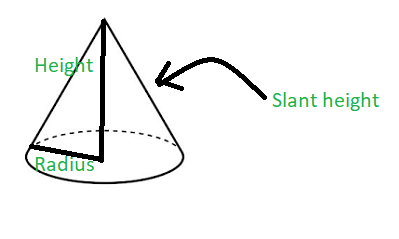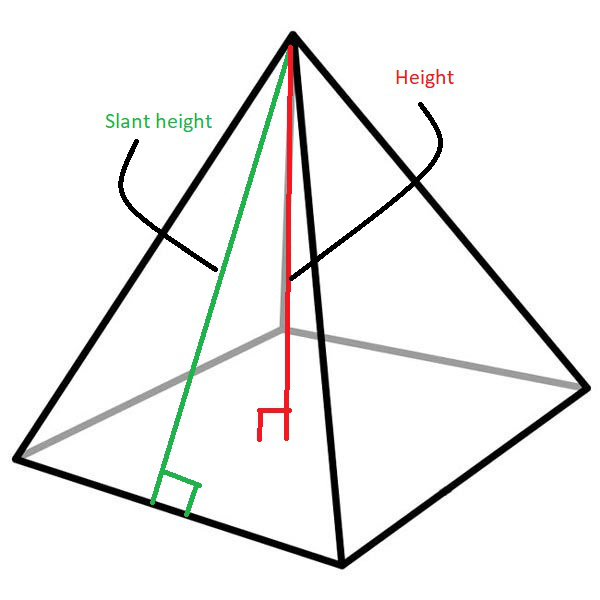# Program to find slant height of cone and pyramid

Given two integers H1 and R representing the height and radius of a cone and two integers H2 and S representing the height and length of base of a pyramid, the task is to find the slant height of the cone and the pyramid.

Examples:

Input: H1 = 4.5, R = 6, H2 = 4, S = 4.8
Output:
Slant height of cone is: 7.5
Slant height of pyramid is: 4.66476

Input: H1 = 2, R = 4, H2 = 4, S = 8
Output:
Slant height of cone is: 4.47214
Slant height of pyramid is: 5.65685

Approach: The slant height of an object such as a cone or a pyramid is the distance measured from any vertex along a lateral face to the base (along the center of the face). The slant height of a right circular cone is uniform throughout the surface and is given by the formula:where,
L is the slant height of the right circular cone
R is the radius of the right circular cone and
H is the height of the right circular cone

The slant height of a pyramid is given by the formula:where,
L is the slant height of the pyramid
S is the side of the base of the pyramid
H is the height of the pyramid

Below is the implementation of the above approach:

## C++

 // C++ program for the above approach #include  using namespace std;   // Function to calculate slant // height of a cone void coneSlantHeight(double cone_h,                      double cone_r) {     // Store the slant height of cone     double slant_height_cone         = sqrt(pow(cone_h, 2)                + pow(cone_r, 2));       // Print the result     cout << "Slant height of cone is: "          << slant_height_cone << '\n'; }   // Function to find the slant // height of a pyramid void pyramidSlantHeight(double pyramid_h,                         double pyramid_s) {       // Store the slant height of pyramid     double slant_height_pyramid         = sqrt(pow(pyramid_s / 2, 2)                + pow(pyramid_h, 2));       // Print the result     cout << "Slant height of pyramid is: "          << slant_height_pyramid << '\n'; }   // Driver Code int main() {     // Dimensions of Cone     double H1 = 4.5, R = 6;       // Function Call for slant height     // of Cone     coneSlantHeight(H1, R);       // Dimensions of Pyramid     double H2 = 4, S = 4.8;       // Function to calculate     // slant height of a pyramid     pyramidSlantHeight(H2, S);       return 0; }

## Java

 // Java program for the above approach import java.io.*; class GFG  {           // Function to calculate slant     // height of a cone     static void coneSlantHeight(double cone_h,                          double cone_r)     {                 // Store the slant height of cone         double slant_height_cone             = Math.sqrt(Math.pow(cone_h, 2)                    + Math.pow(cone_r, 2));               // Print the result         System.out.println("Slant height of cone is: " +          slant_height_cone);     }           // Function to find the slant     // height of a pyramid     static void pyramidSlantHeight(double pyramid_h,                             double pyramid_s)     {               // Store the slant height of pyramid         double slant_height_pyramid             = Math.sqrt(Math.pow(pyramid_s / 2, 2)                    + Math.pow(pyramid_h, 2));               // Print the result         System.out.println("Slant height of pyramid is: " +          slant_height_pyramid);     }           // Driver Code     public static void main (String[] args)     {                 // Dimensions of Cone         double H1 = 4.5, R = 6;               // Function Call for slant height         // of Cone         coneSlantHeight(H1, R);               // Dimensions of Pyramid         double H2 = 4, S = 4.8;               // Function to calculate         // slant height of a pyramid         pyramidSlantHeight(H2, S);           } }   // This code is contributed by AnkThon

## Python3

 # Python 3 program for the above approach from math import sqrt,pow # Function to calculate slant # height of a cone def coneSlantHeight(cone_h, cone_r):   # Store the slant height of cone   slant_height_cone = sqrt(pow(cone_h, 2) + pow(cone_r, 2))     # Print the result   print("Slant height of cone is:",slant_height_cone)   # Function to find the slant # height of a pyramid def pyramidSlantHeight(pyramid_h, pyramid_s):   # Store the slant height of pyramid   slant_height_pyramid = sqrt(pow(pyramid_s/2, 2) + pow(pyramid_h, 2))      # Print the result   print("Slant height of pyramid is:","{:.5f}".format(slant_height_pyramid))   # Driver Code if __name__ == '__main__':   # Dimensions of Cone   H1 = 4.5   R = 6     # Function Call for slant height   # of Cone   coneSlantHeight(H1, R);     # Dimensions of Pyramid   H2 = 4   S = 4.8     # Function to calculate   # slant height of a pyramid   pyramidSlantHeight(H2, S)

## C#

 // C# program for the above approach using System; public class GFG  {     // Function to calculate slant   // height of a cone   static void coneSlantHeight(double cone_h,                               double cone_r)   {       // Store the slant height of cone     double slant_height_cone       = Math.Sqrt(Math.Pow(cone_h, 2)                   + Math.Pow(cone_r, 2));       // Print the result     Console.WriteLine("Slant height of cone is: " +                        slant_height_cone);   }     // Function to find the slant   // height of a pyramid   static void pyramidSlantHeight(double pyramid_h,                                  double pyramid_s)   {       // Store the slant height of pyramid     double slant_height_pyramid       = Math.Sqrt(Math.Pow(pyramid_s / 2, 2)                   + Math.Pow(pyramid_h, 2));       // Print the result     Console.WriteLine("Slant height of pyramid is: " +                        slant_height_pyramid);   }     // Driver Code   public static void Main (string[] args)   {       // Dimensions of Cone     double H1 = 4.5, R = 6;       // Function Call for slant height     // of Cone     coneSlantHeight(H1, R);       // Dimensions of Pyramid     double H2 = 4, S = 4.8;       // Function to calculate     // slant height of a pyramid     pyramidSlantHeight(H2, S);     } }   // This code is contributed by AnkThon

## Javascript

 

Output:

Slant height of cone is: 7.5
Slant height of pyramid is: 4.66476

Time Complexity: O(sqrt(logH1+logR) + sqrt(logH2+logS))
Auxiliary Space: O(1)

Feeling lost in the world of random DSA topics, wasting time without progress? It's time for a change! Join our DSA course, where we'll guide you on an exciting journey to master DSA efficiently and on schedule.
Ready to dive in? Explore our Free Demo Content and join our DSA course, trusted by over 100,000 geeks!

Previous
Next